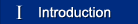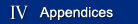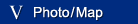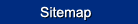go to main contentsHistory

Main Contents begins from here.

# WEIGHTS AND MEASURES

In most parts of the world in 1970, the metric system is the standard system of weight and measures, at least it is legally recognised and used in international trade and industry together with local systems prevailing in each country. In certain countries, the metric system is the standard for all official transactions, notably in the countries of mainland Europe, Scandinavia and Japan. The United States and the United Kingdom legalised the metric system in 1866 and 1897 respectively but both countries retain systems based on the foot and the pound for popular usage and international trade. In Japan, the metric system was adopted in 1893 and in 1924 it became the official system, except in the case of traditional commodities.

The metric system was first proposed in 1670, in France, and until the 19th century the standard was based on one metre being equal to one ten-millionth part of the meridianal quadrant of the earth. The International Bureau of Weights and Measures was established in France in 1876. In 1960, the metric standard was redefined as follows :

1 metre = 1,650,763.73 wavelengths under vacuum of the radiation corresponding to the transition between the energy levels 2p10 and 5d5 of the pure nuclide of Kr86. 0.001m³

The tables of weights and measures given here are those of the metric system and the systems most widely prevailing outside the metric system – those of the United States and the United Kingdom. Also given are weights and measures of Japan which, though archaic, are of interest in this context. The British system (from which the American system is derived) is ancient in origin. The foot was introduced during the Roman occupation and integrated with the inch, a Saxon measure. Standards of volume, such as the bushel, are based on Old English standards of early date.

## THE METRIC SYSTEM

LINEAR WEIGHT CAPACITY
1/1000 millimetre milligram millilitre
1/100 centimetre centigram centilitre
l/10 decimetre decigram decilitre
×1 metre gram(me) litre
×10 decametre decagram decalitre
×100 hectometre hectogram hectolitre
×1000 kilometre kilogram kilolitre
×1 million   metoric ton(ne)

## THE BRITISH SYSTEM

### LINEAR

 1 inch =2.54 centimetres 1 foot =12 inches =0.3048 metre 1 yard =3 feet =0.9144 metre 1 pole =5.5 yards =5.02920 metres 1 chain =22 yards =20.1168 metres 1 furlong =220 yards =201.168 metres 1 mile =1,760 yards =1.609344 kilometres

### WEIGHT (Avoirdupois)

 1 grain =0.06479891 gram 1 dram =27.34 grains =1.77185 grams 1 ounce =16 drams =28.3495 grams 1 pound =16 ounces =0.45359237 kilogram 1 stone =14 pounds =6.35029 kilograms 1 quarter =28 pounds =12.7006 kilograms 1 hundred weight =112 pounds =50.8023 kilograms 1 ton =20 hundred weight =1.01605 metric tons

### CAPACITY

 1 gill =1.42066 decilitres 1 pint =4 gills =0.568262 litre 1 quart =2 pints =1.13652 litre 1 gallon =4 quarts =4.54609 litre 1 peck =2 gallons =9.09218 litre 1 bushel =8 gallons =3.63687 decalitres 1 quarter =8 bushels =2.90950 hectolitres

## THE AMERICAN SYSTEM

### LINEAR

As British system above

### WEIGHT (Avoirdupois)

As British system except the following:

 1 hundredweight = 100 pounds = 45.3592 kilograms 1 ton = 2,000 pounds = 0.907185 metric ton

## CAPACITY

### Liquid

 l gill =0.118294 litre 1 pint =4 gills =0.473176 litre 1 quart =2 pints =0.9463353 litre 1 gallon =4 quarts =3.78541 litre

### Dry

 1 peck =8 dry quarts =8.80978 litre 1 bushel =4 pecks =35.2391 litre

## TRADITIONAL JAPANESE WEIGHTS AND MEASURES

### Linear

 1bu =0.01 shaku =3.03030 millimeters 1 sun =0.1 shaku =3.03030 centimetres 1 shaku =0.303030 metre 1 ken =6 shaku =1.81818 metres 1 jo =10 shaku =3.03030 metres 1 cho =60 ken =109.091 metres 1 ri =36 cho =3.92727 kilometres 1 tan** =26 kujira shaku =9.84848 metres 1 jo** =10 kujira shaku =3.78788 metres

### Weight

 1 monme =3.75 grams 1 kan =1000 monme =3.75 kilograms 1 kin =100 monme =0.600 kilogram

### Capacity

 1 go =0.180391 litres 1 sho =10 go =1.80391 litres 1 to =10 sho =18.0391 litres l koku =10 to =180.391 litres

### Area

 1 tsubo =3.30579 sq.m. 1 se =30 tsubo =99.1736 sq.m. 1 tan =lO se =991.736 sq.m. 1 cho =10 tan =9917.36 sq.m. =2.45063 acres(Br.)

**These measures apply to piece lengths of kimono fabric. Both measures are slightly variable.

Return to top

The contents of this site are excerpted from THE OFFICIAL RECORD OF TIME CAPSULE EXPO'70(March 1975). Please note that company and organization names may differ from those of the current ones.

© Panasonic Corporation 2010